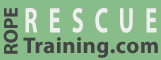# Calculating MA using the T-System

The T-System, which is sometimes referred to as the T-Method or the "adding the tensions" method, is a versatile way to calculate the mechanical advantage of a rope-and-pulley system. Although the T-System may appear complicated, it really isn't. The only math that is required is adding a few one-digit numbers.

The T-System can solve relatively complicated systems including all of the mechanical advantage systems on RopeRescueTraining.com. However, the T-System cannot solve every system imaginable. It works great on simple and compound rigging systems, but it can get overwhelmed by some complex systems.

The T-System always assumes that the pulleys are frictionless and that the ropes make 180-degree turns around the pulleys.

## The Four Steps

The T-System can be completed in four steps:

1. Write "T1" next to the puller.
2. Draw three blank lines next to each pulley.
3. Fill in the blank lines.

### Step 1. Write "T1" next to the puller.

The first step is so easy it barely deserves to be called a "step," but write "T1" next to the rope where the rescuers will be pulling.

### Step 2. Draw three blank lines next to each pulley.

Draw blank lines where the rope enters and exits the pulley, and draw a third blank line near the eye of the pulley. We will use these blank lines to record the T values.

(The "T" in the T-System refers to "tension." We prefix the numbers with a "T" to remind us that we're comparing the tension within the system.)

### Step 3. Fill in the blanks.

This step is a little more complicated:

• Starting at the puller, follow the rope until you reach a pulley.
• Write down the rope's previous T value where the rope enters the pulley. (In this case, the previous T value was the T1 near the hand.)
• Write the same T value where the rope exits the pulley. (The T values entering and exiting pulleys will always be the same.)
• Add the two values together and write this number near the pulley's eye. (In this case, T1 plus T1 is T2.)
• Write this same T value next to the gear that is connected to the pulley's eye (e.g., the load in this example).

Repeat this process by moving down the rope and writing the three T values next to any additional pulleys, until you reach the end of the rope.

### Step 4. Report the mechanical advantage.

The mechanical advantage is the number on the load compared to the number near the puller (which will always be "1"). The load in the above example is labeled T2 so this is a 2:1 system.

## A Two Pulley System

### Steps 1 and 2

The first two steps have been completed in this illustration:

Step 1. Write "T1" next to the puller.

Step 2. Draw three blank lines next to each pulley.

### Steps 3 and 4

Step 3 is a little more complicated.

The pulley nearest the hand was labeled in the same manner as in the previous example: The rope entering the pulley was labeled with the previous T value (T1, from the hand), the rope exiting the pulley was given the same value (T1), and the pulley's eye was labeled with the sum of the two ropes (T2).

Per the rules, we labeled the carabiner and Prusik with the same value as the pulley's eye (T2). (We labeled the values that aren't associated with pulleys in red.)

As we continued along the rope, the upper pulley and anchor were labeled in a similar manner (T1, T1, and T2).

Here's where it gets a little tricky. The T2 from the Prusik and the T1 from the rope combine to T3 just below the Prusik, so there is T3 at the load (i.e., a 3:1 system).

Don't assume that the value entering and exiting pulleys will always be T1 or that the value at the eye of the pulley will always be T2. Yes, this has been the case in these examples, but it won't always be the case.

## Multiple Ropes

If the mechanical advantage system has more than one rope, first label the T values on the rope that is being pulled by the rescuers (the yellow rope in this example). Then label the T values on the next rope, beginning where it is being pulled by the first rope.

In this illustration, we first labeled the T values of the yellow rope (with black text) and then labeled the T values for the red rope (with red text). It is important to notice that the first value on the red rope (just below the knot) was labeled T2, because the eye of the upper pulley has a value of T2.

This is a 4:1 system, because the T value at the load is T4.